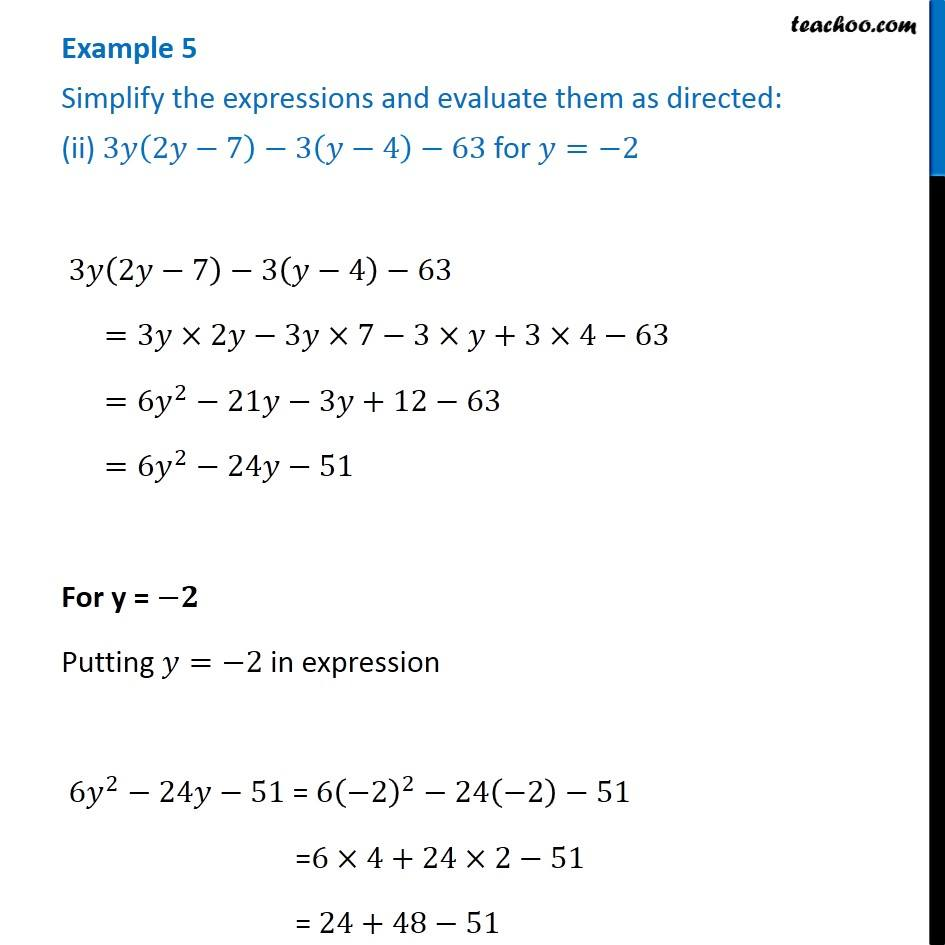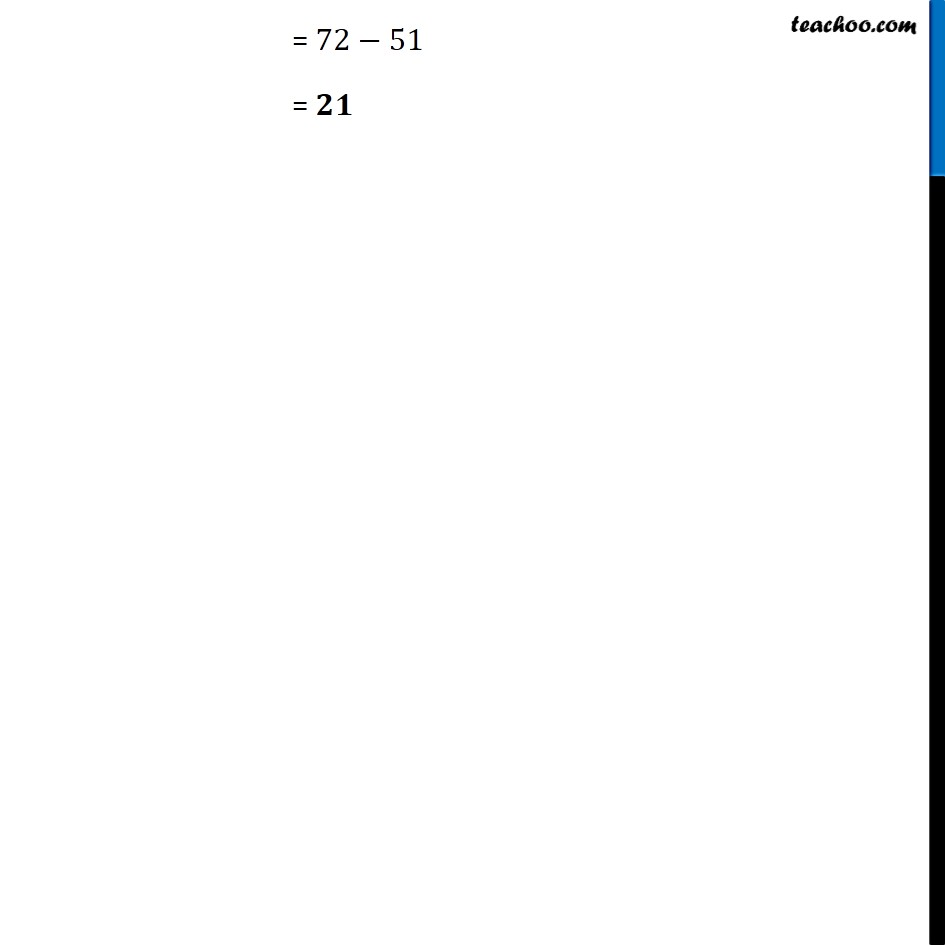Examples

Chapter 8 Class 8 Algebraic Expressions and Identities
Serial order wiseLearn in your speed, with individual attention - Teachoo Maths 1-on-1 Class

### Transcript

Example 5 Simplify the expressions and evaluate them as directed: (ii) 3𝑦(2𝑦−7)−3(𝑦−4)−63 for 𝑦=−2 3𝑦(2𝑦−7)−3(𝑦−4)−63 =3𝑦×2𝑦−3𝑦×7−3×𝑦+3×4−63 =6𝑦^2−21𝑦−3𝑦+12−63 =6𝑦^2−24𝑦−51 For y = −𝟐 Putting 𝑦=−2 in expression 6𝑦^2−24𝑦−51 = 6(−2)^2−24(−2)−51 =6×4+24×2−51 = 24+48−51 = 72−51 = 𝟐𝟏